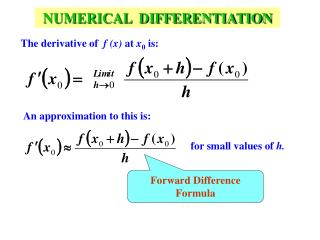DownloadDownload PresentationNUMERICAL DIFFERENTIATION

# NUMERICAL DIFFERENTIATION

Download Presentation## NUMERICAL DIFFERENTIATION

- - - - - - - - - - - - - - - - - - - - - - - - - - - E N D - - - - - - - - - - - - - - - - - - - - - - - - - - -
##### Presentation Transcript

1. NUMERICAL DIFFERENTIATION The derivative of f(x) at x0 is: An approximation to this is: for small values of h. Forward Difference Formula

2. Find an approximate value for The exact value of

3. Assume that a function goes through three points: Lagrange Interpolating Polynomial

4. If the points are equally spaced, i.e.,

5. Three-point formula:

6. If the points are equally spaced with x0in the middle:

7. Another Three-point formula:

8. .............. (1) Alternate approach (Error estimate) Take Taylor series expansion of f(x+h) about x:

9. Forward Difference Formula

10. ................. (2)

11. ................. (2) .............. (1) 2 X Eqn. (1) – Eqn. (2)

12. Three-point Formula

13. The Second Three-point Formula Take Taylor series expansion of f(x+h) about x: Take Taylor series expansion of f(x-h) about x: Subtract one expression from another

14. Second Three-point Formula

15. Forward Difference Formula Error term Summary of Errors

16. First Three-point Formula Error term Summary of Errors continued

17. Second Three-point Formula Error term Summary of Errors continued

18. Example: Find the approximate value of with

19. Using the Forward Difference formula:

20. Using the 1st Three-point formula:

21. Using the 2nd Three-point formula: The exact value of

22. The exact value of is 22.167168 Comparison of the results with h = 0.1

23. Second-order Derivative Add these two equations.

24. NUMERICAL INTEGRATION area under the curve f(x) between In many cases a mathematical expression for f(x) is unknown and in some cases even if f(x) is known its complex form makes it difficult to perform the integration.

25. Area of the trapezoid The length of the two parallel sides of the trapezoid are: f(a) and f(b) The height isb-a

26. Simpson’s Rule:

27. Composite Numerical Integration

28. Riemann Sum The area under the curve is subdivided into n subintervals. Each subinterval is treated as a rectangle. The area of all subintervals are added to determine the area under the curve. There are several variations of Riemann sum as applied to composite integration.

29. In Left Riemann sum, the left-side sample of the function is used as the height of the individual rectangle.

30. In Right Riemann sum, the right-side sample of the function is used as the height of the individual rectangle.

31. In the Midpoint Rule, the sample at the middle of the subinterval is used as the height of the individual rectangle.

32. Composite Trapezoidal Rule: Divide the interval into n subintervals and apply Trapezoidal Rule in each subinterval. where

33. Find by dividing the interval into 20 subintervals.

34. Composite Simpson’s Rule: Divide the interval into n subintervals and apply Simpson’s Rule on each consecutive pair of subinterval. Note that n must be even.

35. where Find by dividing the interval into 20 subintervals.